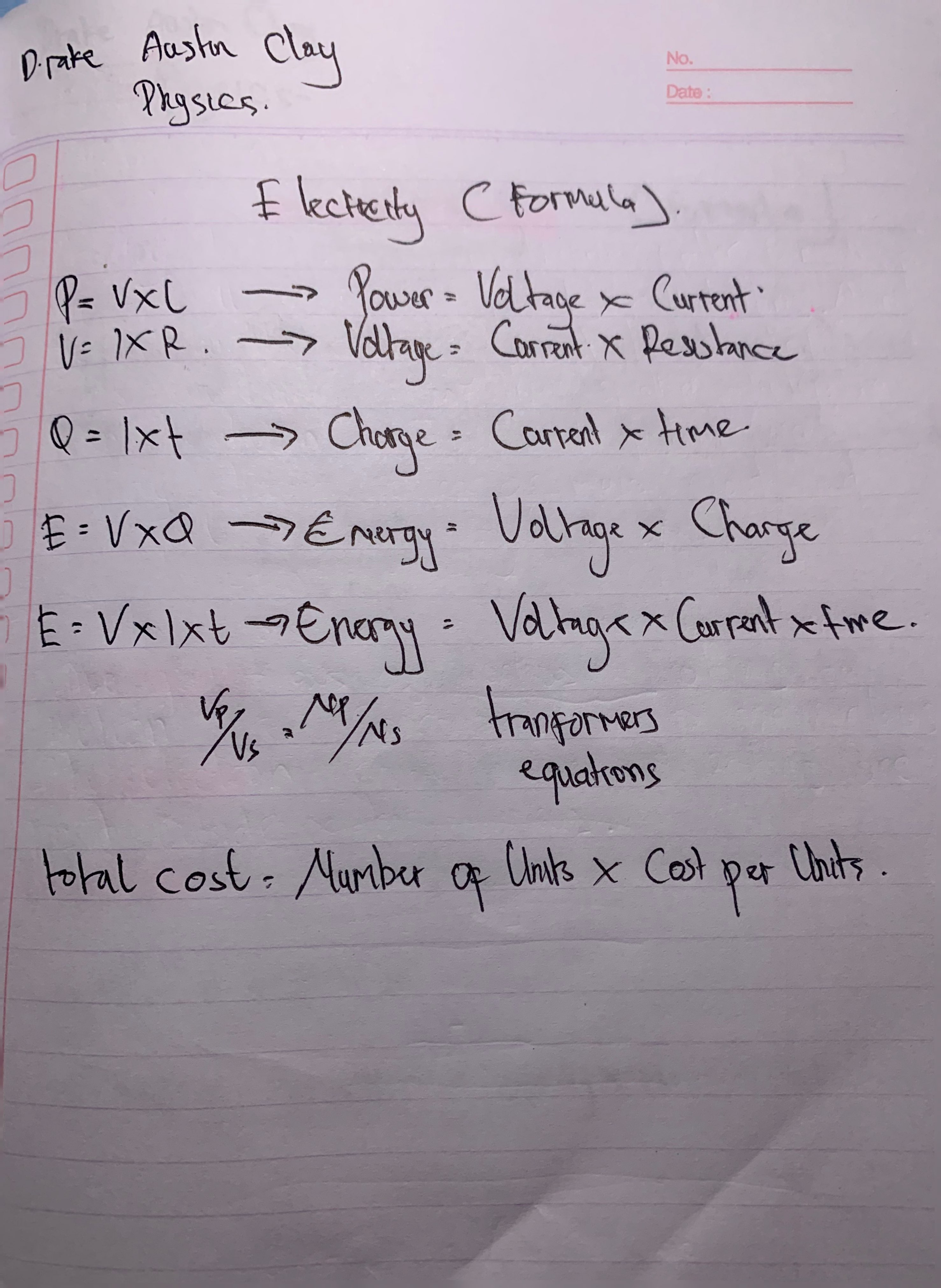Electrycity (Formula)D.rake Austin Clay

No.

Physics.

Date:

E lectecity C Formala).

P= = VxL

Power = Voltage x Current

V= IXR.

Voltage = Current X Resistance

Q = 1xt

Charge : = Carrent x time

E= VxQ

Energy= Voltage x Charge

E = Vxlxt

Energy : Valtage X Current xtre.

Valis

N/Ns

tranformers

equations

total cost = Mumber of Units x Cost per Units.

of 1